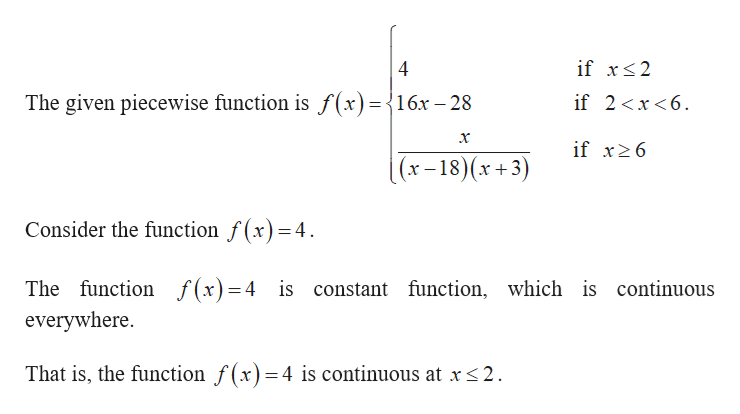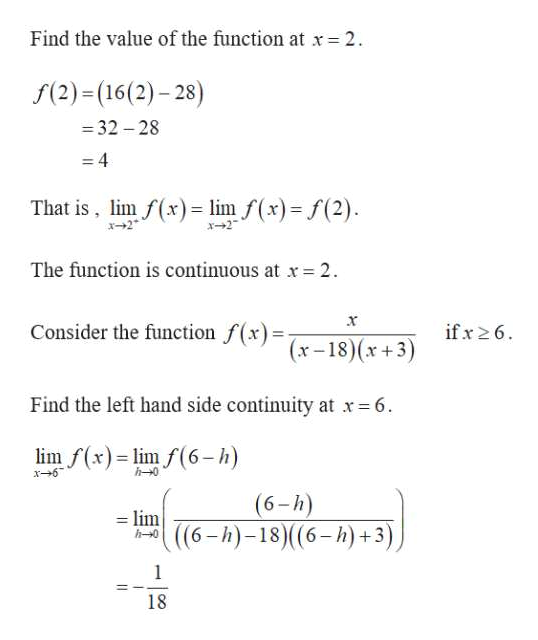# Determine the values of x for which f(x) is discontinuous. (Enter your answers as a comma-separated list. If an answer does not exist, enter DNE.)f(x) =  4   ifx ≤ 216x − 28  if 2 < x < 6 x(x − 18)(x + 3) ifx ≥ 6x =

Question
2 views

Determine the values of x for which f(x) is discontinuous. (Enter your answers as a comma-separated list. If an answer does not exist, enter DNE.)

f(x) =
4     if x ≤ 2
16x − 28    if  2 < x < 6

 x (x − 18)(x + 3)
if x ≥ 6

x =

check_circle

Step 1help_outlineImage Transcriptioncloseif xs2 4 The given piecewise function is f(x)= {16x-28 if 2x<6 if x6 (x-18)(x+3) Consider the function f(x)=4. The function f(x)=4 is constant function, which is continuous everywhere. That is, the function f(x)=4 is continuous at x <2 fullscreen
Step 2
Step 3help_outlineImage TranscriptioncloseFind the value of the function at x 2 f(2) (16(2)-28) -32-28 - 4 That is, lim f(x)= lim f(x)= f(2) x2 The function is continuous at x 2 Consider the function f(x)x-18)(x+3) if x 26 Find the left hand side continuity at x6 lim f(x)lim f(6-h) h-0 x6 (6-h) i(6-h)-18)((6-h)+3) h-0 1 18 fullscreen

### Want to see the full answer?

See Solution

#### Want to see this answer and more?

Solutions are written by subject experts who are available 24/7. Questions are typically answered within 1 hour.*

See Solution
*Response times may vary by subject and question.
Tagged in

### Other